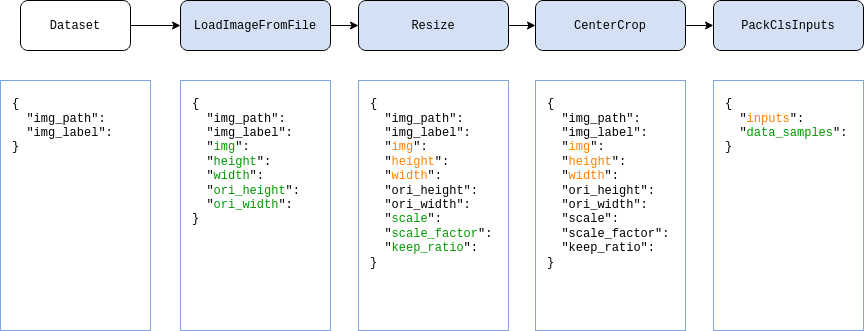Shortcuts

# Data transform¶

In the OpenMMLab repositories, dataset construction and data preparation are decoupled from each other. Usually, the dataset construction only parses the dataset and records the basic information of each sample, while the data preparation is performed by a series of data transforms, such as data loading, preprocessing, and formatting based on the basic information of the samples.

## To use Data Transforms¶

In MMEngine, we use various callable data transforms classes to perform data manipulation. These data transformation classes can accept several configuration parameters for instantiation and then process the input data dictionary by calling. Also, all data transforms accept a dictionary as input and output the processed data as a dictionary. A simple example is as belows:

Note

In MMEngine, we don’t have the implementations of data transforms. you can find the base data transform class and many other data transforms in MMCV. So you need to install MMCV before learning this tutorial, see the MMCV installation guide.

>>> import numpy as np
>>> from mmcv.transforms import Resize
>>>
>>> transform = Resize(scale=(224, 224))
>>> data_dict = {'img': np.random.rand(256, 256, 3)}
>>> data_dict = transform(data_dict)
>>> print(data_dict['img'].shape)
(224, 224, 3)


## To use in Config Files¶

In config files, we can compose multiple data transforms as a list, called a data pipeline. And the data pipeline is an argument of the dataset.

Usually, a data pipeline consists of the following parts:

1. Data loading, use LoadImageFromFile to load image files.

2. Label loading, use LoadAnnotations to load the bboxes, semantic segmentation and keypoint annotations.

3. Data processing and augmentation, like RandomResize.

4. Data formatting, we use different data transforms for different tasks. And the data transform for specified task is implemented in the corresponding repository. For example, the data formatting transform for image classification task is PackClsInputs and it’s in MMPretrain.

Here, taking the classification task as an example, we show a typical data pipeline in the figure below. For each sample, the basic information stored in the dataset is a dictionary as shown on the far left side of the figure, after which, every blue block represents a data transform, and in every data transform, we add some new fields (marked in green) or update some existing fields (marked in orange) in the data dictionary.If want to use the above data pipeline in our config file, use the below settings:

test_dataloader = dict(
batch_size=32,
dataset=dict(
type='ImageNet',
data_root='data/imagenet',
pipeline = [
dict(type='Resize', size=256, keep_ratio=True),
dict(type='CenterCrop', crop_size=224),
dict(type='PackClsInputs'),
]
)
)


## Common Data Transforms¶

According to the functionality, the data transform classes can be divided into data loading, data pre-processing & augmentation and data formatting.

To support loading large-scale dataset, usually we won’t load all dense data during dataset construction, but only load the file path of these data. Therefore, we need to load these data in the data pipeline.

Data Transforms

Functionality

LoadImageFromFile

Load images according to the path.

LoadAnnotations

Load and format annotations information, including bbox, segmentation map and others.

### Data Pre-processing & Augmentation¶

Data transforms for pre-processing and augmentation usually manipulate the image and annotation data, like cropping, padding, resizing and others.

Data Transforms

Functionality

Pad

CenterCrop

Crop the image and keep the center part.

Normalize

Normalize the image pixels.

Resize

Resize images to the specified scale or ratio.

RandomResize

Resize images to a random scale in the specified range.

RandomChoiceResize

Resize images to a random scale from several specified scales.

RandomGrayscale

Randomly grayscale images.

RandomFlip

Randomly flip images.

### Data Formatting¶

Data formatting transforms will convert the data to some specified type.

Data Transforms

Functionality

ToTensor

Convert the data of specified field to torch.Tensor

ImageToTensor

Convert images to torch.Tensor in PyTorch format.

## Custom Data Transform Classes¶

To implement a new data transform class, the class needs to inherit BaseTransform and implement transform method. Here, we use a simple flip transforms (MyFlip) as example:

import random
import mmcv
from mmcv.transforms import BaseTransform, TRANSFORMS

@TRANSFORMS.register_module()
class MyFlip(BaseTransform):
def __init__(self, direction: str):
super().__init__()
self.direction = direction

def transform(self, results: dict) -> dict:
img = results['img']
results['img'] = mmcv.imflip(img, direction=self.direction)
return results


Then, we can instantiate a MyFlip object and use it to process our data dictionary.

import numpy as np

transform = MyFlip(direction='horizontal')
data_dict = {'img': np.random.rand(224, 224, 3)}
data_dict = transform(data_dict)
processed_img = data_dict['img']


Or, use it in the data pipeline by modifying our config file:

pipeline = [
...
dict(type='MyFlip', direction='horizontal'),
...
]


Please note that to use the class in our config file, we need to confirm the MyFlip class will be imported during running.

© Copyright 2022, mmengine contributors. Revision c863e8b1.

Built with Sphinx using a theme provided by Read the Docs.
Versions
latest
stable
v0.8.5
v0.8.4
v0.8.3
v0.8.2
v0.8.1
v0.8.0
v0.7.4
v0.7.3
v0.7.2
v0.7.1
v0.7.0
v0.6.0
v0.5.0
v0.4.0
v0.3.0
v0.2.0